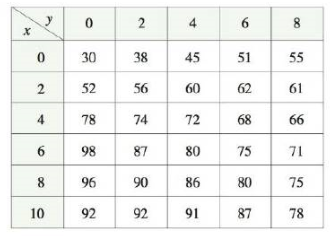Chapter 14, Problem 12RE

Chapter
Section
Textbook Problem

Find a linear approximation to the temperature function T(x, y) in Exercise 11 near the point (6, 4). Then use it to estimate the temperature at the point (5, 3.8).To determine

To find: The linearzation of the temperature function T(x,y) near the point (6,4) , use this approximation and approximate the value of T(x,y) at the point (5,3.8) .

Explanation

Given:

The function is, T(x,y) .

The values of Tx(6,4)3.5andTy(6,4)3.0 .

Calculation:

The linear approximation of the function T(x,y) at (6,4) is defined as,

T(6,4)T(6,4)+Tx(6,4)(x6)+Ty(6,4)(y4) (1)

Substitute the respective values in the equation (1) and find the linear approximation,

T(6,4)T(6,4)+Tx(6,4)(x6)+Ty(6,4)(y4)T(6,4)80+3

Still sussing out bartleby?

Check out a sample textbook solution.

See a sample solution

The Solution to Your Study Problems

Bartleby provides explanations to thousands of textbook problems written by our experts, many with advanced degrees!

Get Started

In Exercises 7-12, solve for y in terms of x. 2x+y6=11

Calculus: An Applied Approach (MindTap Course List)

In Exercises 1728, use the logarithm identities to obtain the missing quantity.

Finite Mathematics and Applied Calculus (MindTap Course List)

Convert the following percents to decimals. 3. 59.68%

Contemporary Mathematics for Business & Consumers

Find each product: (4xy2z3)(4x5z3)

Elementary Technical Mathematics

In Problems 41-43, solve each system of equations using inverse matrices. 42. (See Problem 40.)

Mathematical Applications for the Management, Life, and Social Sciences